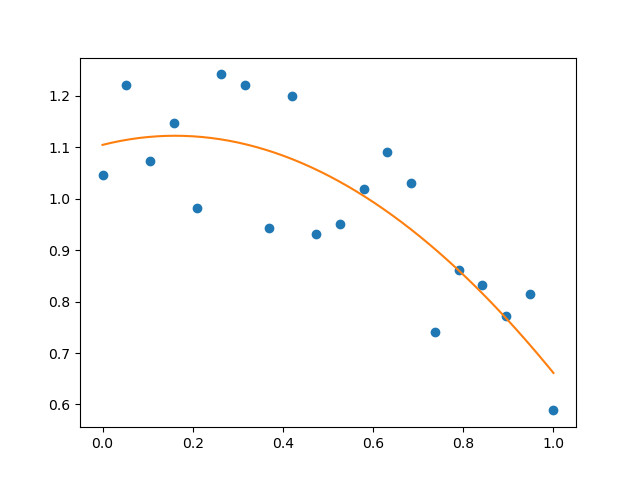# Fitting to polynomial¶

Plot noisy data and their polynomial fit```import numpy as np
import matplotlib.pyplot as plt

np.random.seed(12)

x = np.linspace(0, 1, 20)
y = np.cos(x) + 0.3*np.random.rand(20)
p = np.poly1d(np.polyfit(x, y, 3))

t = np.linspace(0, 1, 200)
plt.plot(x, y, 'o', t, p(t), '-')
plt.show()
```

Total running time of the script: ( 0 minutes 0.028 seconds)

Gallery generated by Sphinx-Gallery